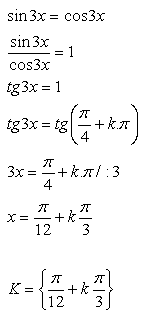sk|cz|

# Linear Goniometric Equations

1.Fill the following table of chosen goniometric functions: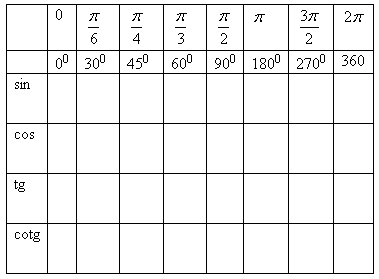Solution: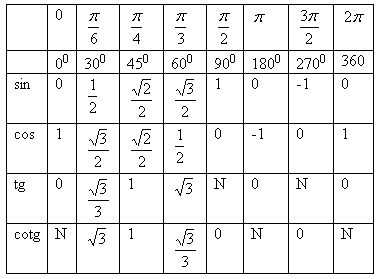2.Fill the table of signs for different quadrants: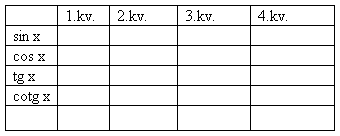Solution: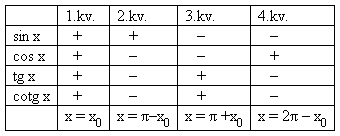3. Solve in real numbers: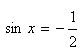Solution: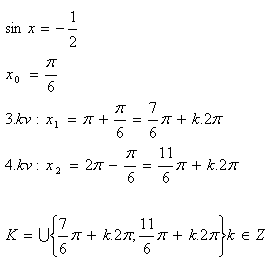4. Solve in real numbers: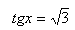Solution: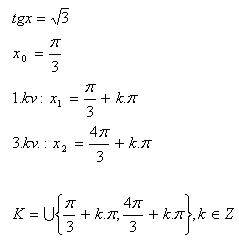5. Solve in real numbers: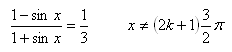Solution: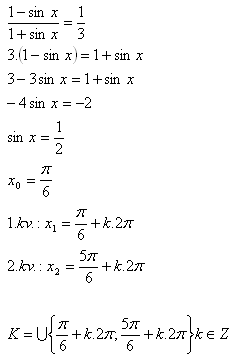6. Solve in real numbers: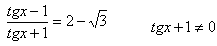Solution: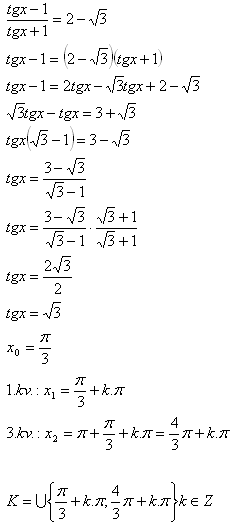7.Solve in real numbers: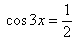Solution: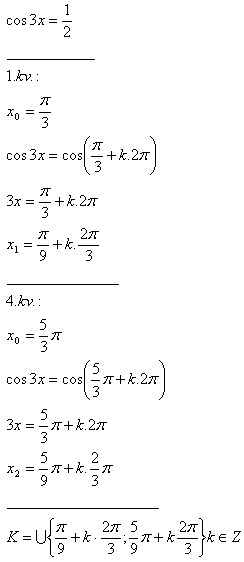8.Solve in real numbers: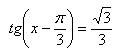Solution: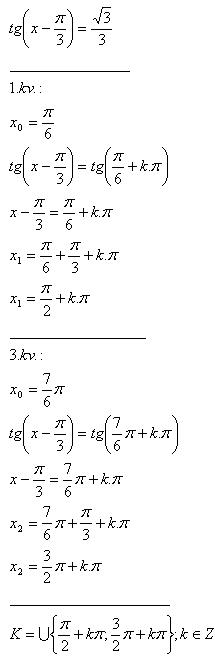9.Solve in real numbers: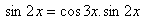Solution: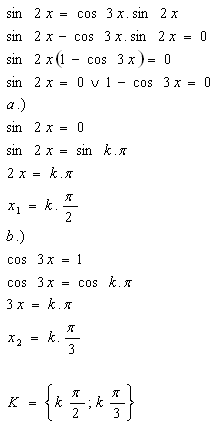10.Solve in real numbers: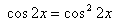Solution: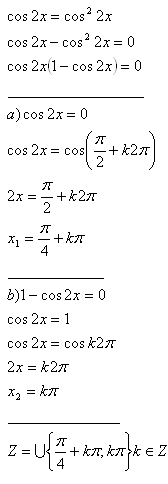11.Solve in real numbers: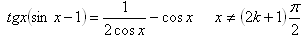Solution: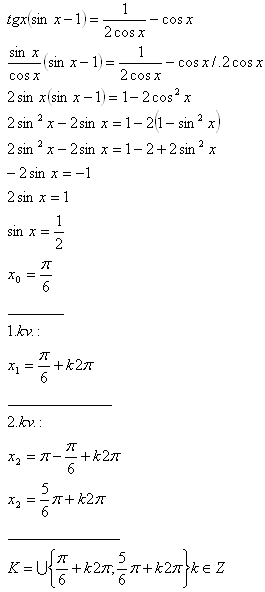12.Solve in real numbers: sin 3x = cos 3x.

Solution: